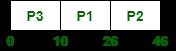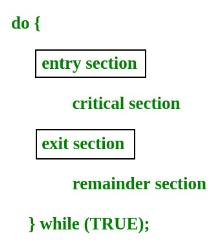Courses

# Test: Process Synchronization- 1

## 11 Questions MCQ Test Operating System | Test: Process Synchronization- 1

Description
This mock test of Test: Process Synchronization- 1 for Computer Science Engineering (CSE) helps you for every Computer Science Engineering (CSE) entrance exam. This contains 11 Multiple Choice Questions for Computer Science Engineering (CSE) Test: Process Synchronization- 1 (mcq) to study with solutions a complete question bank. The solved questions answers in this Test: Process Synchronization- 1 quiz give you a good mix of easy questions and tough questions. Computer Science Engineering (CSE) students definitely take this Test: Process Synchronization- 1 exercise for a better result in the exam. You can find other Test: Process Synchronization- 1 extra questions, long questions & short questions for Computer Science Engineering (CSE) on EduRev as well by searching above.
QUESTION: 1

### Consider the following code fragment: if (fork() == 0) { a = a + 5; printf("%d,%dn", a, &a); } else { a = a –5; printf("%d, %dn", a, &a); } Let u, v be the values printed by the parent process, and x, y be the values printed by the child process. Which one of the following is TRUE?

Solution:

fork() returns 0 in child process and process ID of child process in parent process. In Child (x), a = a + 5 In Parent (u), a = a – 5; Therefore x = u + 10. The physical addresses of ‘a’ in parent and child must be different. But our program accesses virtual addresses (assuming we are running on an OS that uses virtual memory). The child process gets an exact copy of parent process and virtual address of ‘a’ doesn’t change in child process. Therefore, we get same addresses in both parent and child. See this run for example.

QUESTION: 2

### Three concurrent processes X, Y, and Z execute three different code segments that access and update certain shared variables. Process X executes the P operation (i.e., wait) on semaphores a, b and c; process Y executes the P operation on semaphores b, c and d; process Z executes the P operation on semaphores c, d, and a before entering the respective code segments. After completing the execution of its code segment, each process invokes the V operation (i.e., signal) on its three semaphores. All semaphores are binary semaphores initialized to one. Which one of the following represents a deadlockfree order of invoking the P operations by the processes?

Solution:

Option A can cause deadlock. Imagine a situation process X has acquired a, process Y has acquired b and process Z has acquired c and d. There is circular wait now. Option C can also cause deadlock. Imagine a situation process X has acquired b, process Y has acquired c and process Z has acquired a. There is circular wait now. Option D can also cause deadlock. Imagine a situation process X has acquired a and b, process Y has acquired c. X and Y circularly waiting for each other.

Consider option A) for example here all 3 processes are concurrent so X will get semaphore a, Y will get b and Z will get c, now X is blocked for b, Y is blocked for c, Z gets d and blocked for a. Thus it will lead to deadlock. Similarly one can figure out that for B) completion order is Z,X then Y.

QUESTION: 3

### The atomic fetch-and-set x, y instruction unconditionally sets the memory location x to 1 and fetches the old value of x in y without allowing any intervening access to the memory location x. consider the following implementation of P and V functions on a binary semaphore . void P (binary_semaphore *s) {   unsigned y;   unsigned *x = &(s->value);   do {      fetch-and-set x, y;   } while (y); } void V (binary_semaphore *s) {   S->value = 0; } Which one of the following is true?

Solution:

Let us talk about the operation P(). It stores the value of s in x, then it fetches the old value of x, stores it in y and sets x as 1. The while loop of a process will continue forever if some other process doesn't execute V() and sets the value of s as 0. If context switching is disabled in P, the while loop will run forever as no other process will be able to execute V().

QUESTION: 4

A shared variable x, initialized to zero, is operated on by four concurrent processes W, X, Y, Z as follows. Each of the processes W and X reads x from memory, increments by one, stores it to memory, and then terminates. Each of the processes Y and Z reads x from memory, decrements by two, stores it to memory, and then terminates. Each process before reading x invokes the P operation (i.e., wait) on a counting semaphore S and invokes the V operation (i.e., signal) on the semaphore S after storing x to memory. Semaphore S is initialized to two. What is the maximum possible value of x after all processes complete execution?

Solution:

Processes can run in many ways, below is one of the cases in which x attains max value

Semaphore S is initialized to 2

Process W executes S=1, x=1 but it doesn't update the x variable.

Then process Y executes S=0, it decrements x, now x= -2 and signal semaphore S=1

Now process Z executes s=0, x=-4, signal semaphore S=1
Now process W updates x=1, S=2 Then process X executes X=2
So correct option is D

QUESTION: 5

Three processes arrive at time zero with CPU bursts of 16, 20 and 10 milliseconds. If the scheduler has prior knowledge about the length of the CPU bursts, the minimum achievable average waiting time for these three processes in a non-preemptive scheduler (rounded to nearest integer) is _____________ milliseconds.

Solution:

Use SRTF, for the minimum achievable average waiting time : Gantt chart isSince, TAT = CT - AT and WT = TAT - BT, so WT = CT - AT - BT = CT - (AT+BT) Therefore, Avg, WT = {(26-0-16) + (46-0-20) + (10-0-10)} / 3 = {10 + 26 + 0} / 3 = 36 / 3 = 12

QUESTION: 6

On a system using non-preemptive scheduling, processes with expected run times of 5, 18, 9 and 12 are in the ready queue. In what order should they be run to minimize wait time?

Solution:

The processes should execute in SJF manner to get the lowest waiting time. So, the order should be 5, 9, 12, 18. Option (B) is correct.

QUESTION: 7

Feedback queues

Solution:

Multilevel Feedback Queue Scheduling (MLFQ) keep analyzing the behavior (time of execution) of processes and according to which it changes its priority of execution of processes. Option (C) is correct.

QUESTION: 8

In a dot matrix printer the time to print a character is 6 m.sec., time to space in between characters is 2 m.sec., and the number of characters in a line are 200. The printing speed of the dot matrix printer in characters per second and the time to print a character line are given by which of the following options?

Solution:

Total no of characters = 200 (each character having one space) Time taken to print one character = 6 ms; Time taken to print one space = 2 ms. character printing = 200 * 6 = 1200 ms space printing = 200 * 2 = 400 ms total printing time = 1200 + 400 = 1600 ms = 1.6 s. The printing speed of the dot matrix printer in characters per second = 200 / 1.6 = 125 / s. So, option (E) is correct.

QUESTION: 9

For switching from a CPU user mode to the supervisor mode following type of interrupt is most appropriate

Solution:

For switching from a CPU user mode to the supervisor mode Software interrupts occurs. Software interrupts is internal interrupt triggered by some some software instruction. And external interrupt is caused by some hardware module. Option (C) is correct.

QUESTION: 10

The following C program

main()
{
fork() ; fork() ; printf ("yes");
}
If we execute this core segment, how many times the string yes will be printed ?

Solution:

Number of times YES printed is equal to number of process created. Total Number of Processes = 2n where n is number of fork system calls. So here n = 2, 24 = 4
fork ();   // Line 1
fork ();   // Line 2

So, there are total 4 processes (3 new child processes and one original process). Option (C) is correct.

QUESTION: 11

Which of the following need not necessarily be saved on a context switch between processes?

Solution:

The values stored in registers, stack pointers and program counters are saved on context switch between the processes so as to resume the execution of the process. There's no need of saving the contents of TLB as it is invalidated after each context switch. So, option (B) is correct

Track your progress, build streaks, highlight & save important lessons and more!

### Similar Content### Related tests